# Plotluck - “I’m feeling lucky” for ggplot

## Motivation

Generally, in statistical plotting software we distinguish low-level functions (drawing points, lines, labelling axes) and higher-level interfaces to draw certain kinds of diagrams, taking care of many details by itself. In R, a number of packages have been developed on top of base graphics, such as grid, lattice, and the popular ggplot2 package. The current package builds on top of the latter one, but even goes a step further. We are aiming at an abstraction level of visualization where the user only specifies the “what” (data and variable relations) and leaves all other decisions about the “how” (e.g., choice of the type of diagram) to the software.

Let’s take the well-known iris dataset as an example. Say we are interested in the relation of petal length and petal width for different species. Both these variables are numeric, so a scatter plot might be a good representation. Also, it is often a good visual aid to show an approximating smoothing line. There are only three different species in the data set, so we won’t overcrowd the graph by using different colors. Given these considerations, we might write the following lines:

library(ggplot2)
data(iris)
ggplot(iris, aes(x=Petal.Length, y=Petal.Width, color=Species)) + geom_point() + geom_smooth()
#> geom_smooth() using method = 'loess'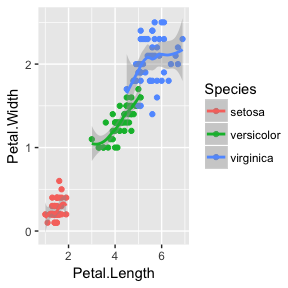As we see, ggplot2 makes it quite easy to create a plot. However, we still have to think about representation, which type of plot to use, and through which aesthetics to express variables. What if we could just concentrate on the relation we want to visualize? The following is the equivalent in plotluck:

library(plotluck)
plotluck(iris, Petal.Width~Petal.Length|Species)
#> geom_smooth() using method = 'loess'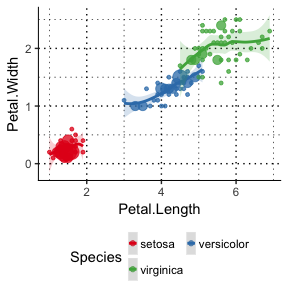Admittedly in this simple example there is not a lot of reduction in the amount of typing; however, in real-world scenarios often more thought and preprocessing is necessary. For mixed data types, the best plot type is not always as obvious. You might also want to quickly visualize two variables without knowing their type beforehand - especially if the data contains a large number of variables or you make a trellis plot of all of them (see below for an example).

After looking at a graph for the first time, you might notice that outliers make it hard to see most of the data, so you plot it again using a logarithmic axis transform. If a factor has a large number of levels, it can help to sort them first by the value of the dependent variable, or cluster many infrequent levels into a single default value. You want to make sure that observation weights and missing values are accounted for accurately.

Plotluck is a tool for exploratory data visualization in R that automates such steps. Similarly as going from base graphics to higher level functions, it simplifies and speeds up the process of data visualization; equally similar, this comes at the price of a reduced flexibility in specializing and “tweaking” individual plots.

## A Longer Example

Let us eplore Hans Rosling’s famous gapminder data . At first, we might just want to know what variables it contains and how they are distributed. In plotluck, the dot symbols stands for “all variables in the data set”:

library(gapminder)
plotluck(gapminder, .~1)
#> Factor variable country has too many levels (142), truncating to 30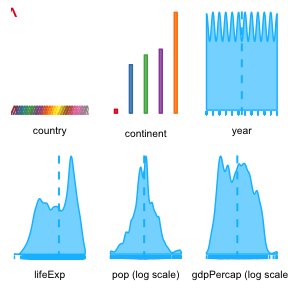In this grid view, each distribution is represented with a minimal thumbnail picture, but we get a general sense of the data. There are 2 categorical and 4 continuous variables; the amount of data is uniform for a range of years; one continent has very few, another one very many rows; pop and gdpPercap have very skewed distributions, so a log-transform has been applied.

Say we are now interested in explanatory variables for target lifeExp. It seems reasonable to weight graphs by population in the following. Switching the verbose=TRUE option on, plotluck will print some information about its decisions.

opts <- plotluck.options(verbose=TRUE)
plotluck(gapminder, lifeExp~., weights=pop, opts=opts)
#> Plotting lifeExp against each variable
#> Factor variable country has too many levels (142), truncating to 10
#> Ordering variables according to conditional entropy:
#>        var cond.ent
#>    lifeExp 0.000000
#>        pop 2.011591
#>  gdpPercap 2.522763
#>    country 2.529314
#>       year 2.530153
#>  continent 2.727954
#> Factor variable country has too many levels (142), truncating to 30
#> geom_smooth() using method = 'gam'
#> geom_smooth() using method = 'gam'
#> geom_smooth() using method = 'gam'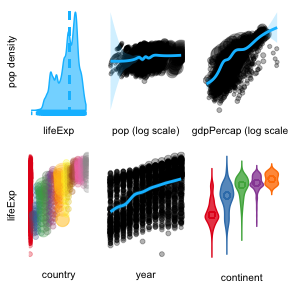As can be seen from the printout, conditional entropies are estimated for ordering the plot (lower values indicate stronger informational value). From the overview, we see a clear correlation of lifeExp with gdpPercap, year, and continent. Lets zoom in on the latter:

plotluck(gapminder, lifeExp~continent, weights=pop, opts=opts)
#> Ordering continent levels by lifeExp
#> Not applying logarithmic axis scaling for lifeExp; expansion ratio is 0.801001, trans.log.thresh = 2.000000
#> Choosing geom='violin' out of possible options: 'violin, box, scatter'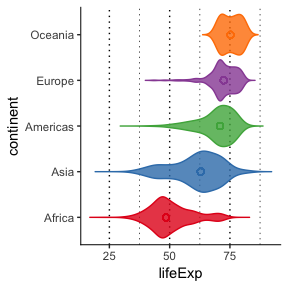The continents are ordered by median lifeExp, fom Africa to Oceania. The spread is also largest for Africa. Which countries are actually accounted for per continent?

plotluck(gapminder, lifeExp~continent+country, weights=pop, opts=opts)
#> Ordering country levels by lifeExp
#> Ordering continent levels by lifeExp
#> Factor variable country has too many levels (142), truncating to 30
#> Choosing geom='heat' out of possible options: 'spine, heat'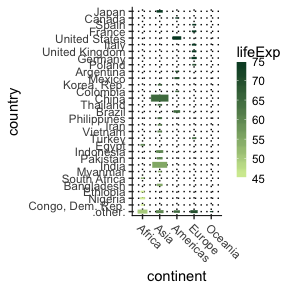Note how in the resulting heat map, rectangles are scaled according to population - this makes China and India stand out.

Another typical question is, how have the distributions changed over time?

plotluck(gapminder, lifeExp~year|continent, weights=pop, opts=opts)
#> Ordering continent levels by lifeExp
#> Not applying logarithmic axis scaling for year; expansion ratio is 0.999321, trans.log.thresh = 2.000000
#> Not applying logarithmic axis scaling for lifeExp; expansion ratio is 0.801099, trans.log.thresh = 2.000000
#> Choosing geom='scatter' out of possible options: 'hex, scatter'
#> geom_smooth() using method = 'loess'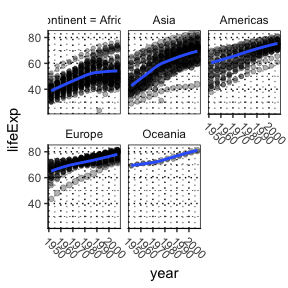It is also possible (and can sometimes be fun) to produce random visualizations from a dataset:

set.seed(6)
sample.plotluck(gapminder, weights=pop, opts)
#> plotluck(gapminder, country ~ gdpPercap, weights = pop,  = opts)
#> Ordering country levels by gdpPercap
#> Factor variable country has too many levels (142), truncating to 30
#> Applying logarithmic axis scaling for gdpPercap; expansion ratio is 4.642230, trans.log.thresh = 2.000000
#> Choosing geom='scatter' out of possible options: 'violin, box, scatter'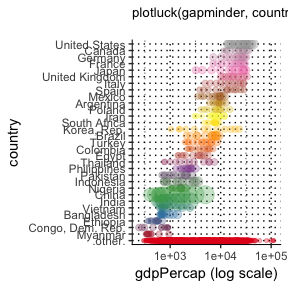## Heuristics for Choosing the Plot Type

For choosing a suitable type of plot, the algorithm takes into account:

• The shape of the user-supplied formula; how many variables, are they explanatory or conditional?
• The type of these variables. Mainly, it distinguishes between numeric (continuous data), ordered (ordinal data), and unordered factors (categorical data). While ordered factors seem to be used somewhat less prominently in applied data analysis, for visualization it is sometimes beneficial to treat them similarly as numeric types, e.g., for sorting other dependent factors.
• Additionally but less importantly, a few heuristics depend on instance counts. E.g., for small data sets, simple scatter plots can reveal more details than density diagrams or histograms; spine plots get confusing with too many factor levels; and if there is only a single data point per factor combination, we would rather see a bar plot than a degenerated box plot.

One-variable numeric (resp. factor) distributions (formulas of the form y~1) are usually represented by density (resp. Cleveland dot) charts.

For two numerical variables (y~x), by default a scatter plot is produced, but for high numbers of points a hexbin is preferred.

The relation between two factor variables can be depicted best by a spine (a.k.a., mosaic) plot; it is very intuitive to see probabilities represented by areas. The only downside is that for three-dimensional spine plots, it is necessary to identify the levels of the second variable (arranged along the y-axis) by counting the rectangles, which becomes unwieldy for more than a handful ones. The fallback then is a heat map.

For a mixed-type (factor/numeric) pair of variables, violin plots are generated. However, if the resulting graph would contain too many of those in a row, the algorithm switches to box plots.

The case of a response with two dependent variables (y~x+z) is covered by either a spine plot (if all are factors) or a heat map.

We attempt to fit conditional variables into the same graph by using coloring; if this is not possible, facetting is applied. Numeric vectors are discretized accordingly. Facets are laid out horizontally or vertically according to the plot type, up to some maximum number of rows and columns.

Some additional elements to plots seem useful more often then not, so they are drawn by default: Density plots and histograms come with an overlaid vertical median line and a rug plot at the border; in violin plots, the median is indicated by a point. Scatter and hexbin plots have a smoothing line and standard deviation. We prefer the more modern violin and density plots to traditional box-and-whisker plots and histograms, as they convey the shape of a distribution better. Box plots show quantiles and outliers, but these are less useful if the distribution is not unimodal. For histograms, a wrong choice of the number of bins can create misleading artifacts.

One common issue in scatter plots is overplotting of duplicate values in the data: the user will only notice a single point. As a remedy, plotluck can use either jittering (randomly shifting points by a small distance), or adjust the point size according to the count (resp., when weights are supplied, the total weight of all data instances represented). The latter representation is the default. Points are also plotted more transparently so that the intersection of nearby points visually leads to darker areas.

Following Cleveland’s advice, factors are plotted preferably on the y-axis to make labels most readable and compact at the same time. Moreover, we prefer dots to bars to maximize the “information content per ink”, unless there are few factor levels and the plot direction is not obvious at first glance.

Generally, from three dimensions on, visualization becomes more challenging. Heat maps are a good compromise in terms of flexibility and robustness; they are applicable both for numeric and categorical data, and can often illustrate trends quite accurately. However, their limitations lie in the necessary discretization on all (the two spatial and the color) axis, and it is good to keep these in mind. The color value represents a ‘central tendency’ of the subset of data instances that are closest to the grid point, but the variation within this bin cannot be shown. If the color represents a numeric variable, the central value is computed as the median; if it is an ordered factor, it is the factor closest to the median, when treating the levels as integers; and for unordered factors, it is the mode (most frequent value). Especially in the last case, this value might not be meaningful if the class distribution is close to uniform.

Rather than completely tiling the grid without spaces, we reduce the size of rectangles in accordance with the total weight or count of data instances. This way, we can incorporate additional coverage information without sacrificing too much of the ‘landscape impression’.

More advanced three-dimensional approximations such as contour plots, 3D bar charts and scatter plots are not supported. While they can be compelling for abstract concepts and smooth mathematical functions, we feel it is very difficult to get them right without careful manual tweaking of perspective and coverage, let alone automatically. For many distributions, there might not exist a good 3D approximation at all.

## Other Features

Besides automated selection of plot type, plotluck offers the following features:

• A relationship with an unordered factor can often become more visible if its levels are sorted, rather than shown in alphabetical order. If the dependent variable is numeric or ordinal, we use it as the sort key; otherwise, we try to use other numeric variables, or just sort by frequency.
• Automatic application of axis scaling, when appropriate (logarithmic or log-modulus). The heuristic is based on the proportion of total display range that is occupied by the ‘core’ region of the distribution between the lower and upper quartiles. We calculate the magnification factor for it under scaling, and decide in favor if it is larger than some threshold.
• Correct handling and visualization of instance weights. All calculations take weights into account when supplied.
• If the data set is too large to plot, sampling is applied.

## Limitations

Plotluck is designed for generic out-of-the-box plotting to support exploratory data analysis. While its design objective is to require as little customization as possible, some aspects that might be subject to individual preferences can be overriden through option parameters. However, plotluck is not suitable to produce specialized types of plots that arise in certain application domains (e.g., association, stem-and-leaf, star plots, geographic maps, etc). It is restricted to at most three variables. Parallel plots with variables on different scales (such as time series of multiple related signals) are not supported either.